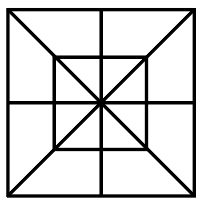Question 1

# How many triangles are there in the following figure ?Solution

count all the squares as shown in the figure

take the highest digit and multiply with 2

(8*2)+(8*2)= 16+16 =32

there are total 32 triangles present in the figure.

• Free SSC Study Material - 18000 Questions
• 230+ SSC previous papers with solutions PDF